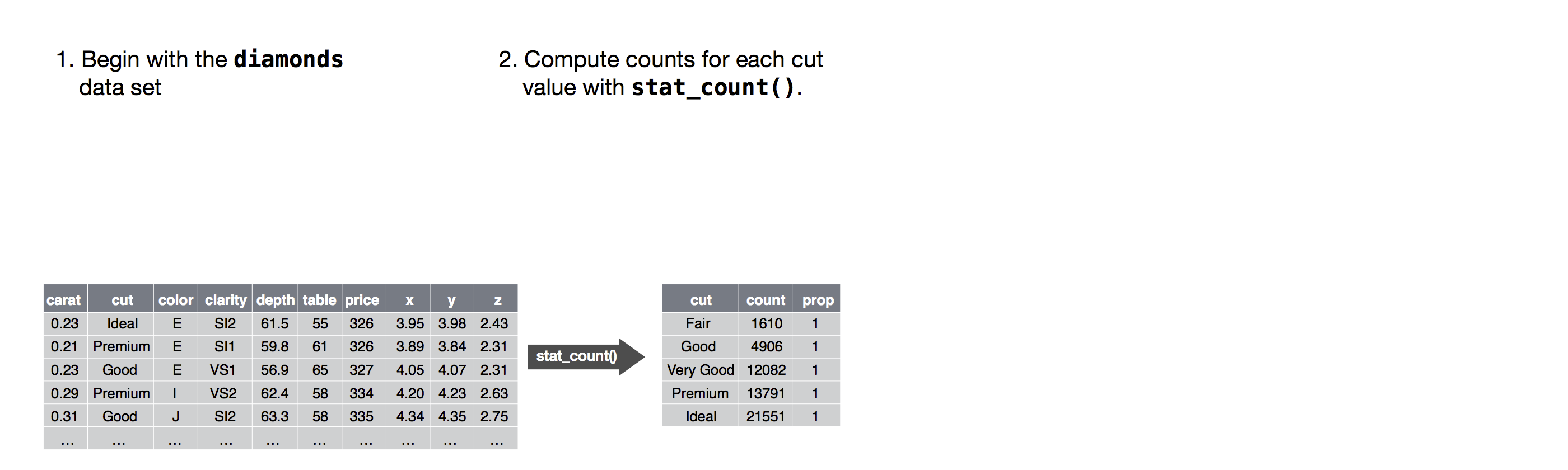## 整体介绍

### 要素

• 数据 (data)
• 映射 (mapping): 建立数据与图形元素的关系
• 几何对象 (geom)：对数据的渲染和展示
• 统计变换 (stats)
• 标度 (scale)
• 坐标系 (coord)
• 分面 (facet)
• 主题 (theme)

ggplot2 is a powerful and a flexible R package, implemented by Hadley Wickham, for producing elegant graphics.

The concept behind ggplot2 divides plot into three different fundamental parts: Plot = data + Aesthetics + Geometry.

The principal components of every plot can be defined as follow:

• data is a data frame （数据分组时必须根据行，而不能根据列）
• Aesthetics is used to indicate x and y variables. It can also be used to control the color, the size or the shape of points, the height of bars, etc….. （映射过程，数据关联到图形，分组）
• Geometry defines the type of graphics (histogram, box plot, line plot, density plot, dot plot, ….)

There are two major functions in ggplot2 package: qplot() and ggplot() functions.

• qplot() stands for quick plot, which can be used to produce easily simple plots.
• ggplot() function is more flexible and robust than qplot for building a plot piece by piece.

### 映射

• 颜色类：color（颜色或边框）、fill（填充）和 alpha（透明度）
• 形状类：linetype（线型）、size（点大小或线宽度）和 shape（形状）
• 位置类：x, y, xmin, xmax, ymin, ymax, xend, yend
• 特殊类：group 和 order；字符串映射

``````ggplot(data = <DATA>, mapping = aes(<MAPPINGS>)) +
<GEOM_FUNCTION>(mapping = aes(<MAPPINGS>))

``````

### 分组

``````p<-ggplot(data = dexp, aes(x = Sample, y = Expression))
p + geom_line(aes(group = Gene, color = Gene)) +
geom_smooth(aes(group = 1))
``````

### 分面

`facet_wrap``facet_grid`

``````facet_wrap(facets, nrow = NULL, ncol = NULL, scales = "fixed",
shrink = TRUE, labeller = "label_value", as.table = TRUE,
switch = NULL, drop = TRUE, dir = "h", strip.position = "top")
``````

• facets: 分面参数如 `~Group`，表示用 Group 变量进行数据分面
• nrow: 绘制图形的行数
• ncol: 绘制图形的列数，一般 nrow/ncol 只设定一个
• scales：
• fixed，小图均使用统一坐标
• free，每个小图按照各自数据范围自由调整坐标
• free_x，自由调整 x 轴刻度范围
• free_y，为自由调整 y 轴刻度范围

facet_grid（可以针对两个变量进行非面）

``````facet_grid(facets, margins = FALSE, scales = "fixed", space = "fixed",
shrink = TRUE, labeller = "label_value", as.table = TRUE,
switch = NULL, drop = TRUE)
``````

• facets: 应用两个标准分面，如 `Gene ~ Group`, 基因分行，group 分列
• margins: Ture，包含所有数据的组
• space: 每张小图的坐标宽度，值同 scales
``````##分面
p<-ggplot(data = dexp, aes(x = Sample, y = Expression))
p + geom_point() +
facet_wrap(~Gene, scales = "free_x", nrow = 5)

dexp_small<-filter(dexp, Gene %in% paste("G", 1:9, sep = ""))
ps<-ggplot(data = dexp_small, aes(x = Sample, y = Expression))
ps + geom_point(aes(color = Length)) +
facet_grid(Gene ~ Group, scales = "free", margins = T,
space = "free")
``````

### 统计变换

ggplot 中和统计变换相关的函数

``````#geom_histogram(
#  stat = "bin",     #数据的统计方式：按窗口统计
#  binwidth = NULL,  #窗口大小
#  bins = NULL,      #分成多少个窗口
#  mapping = NULL,   #y 轴是什么，数目。.count.. 密度。.density..
#)

# 以下两种方法等价

p1 + geom_histogram(binwidth = 200,aes(x = Length, y = ..count..))
p1 + stat_bin(binwidth = 200, aes(x = Length, y = ..count..))

# 我们需要展示出某个变量的某种统计特征的时候，需要用到统计变换，生成变量的名字必须用点号围起来。
``````

### 常用简单图形

• geom_point: 散点图
• geom_bar: 条形图
• geom_line: 折线图（需指定分组信息）
• geom_area: 面积图
• geom_text: 添加标签

• stack 图形元素堆叠
• dodge 图形并排放置
• fill 堆叠图形元素并将高度标准化
• identity 不做调整
• jitter 避免点重合

### 查看具体数据

`ggplot_build()`

### 标度 scale

Scales control the details of how data values are translated to visual properties. Override the default scales to tweak details like the axis labels or legend keys, or to use a completely different translation from data to aesthetic.

``````#name: 修改引导元素名称
p + scale_x_discrete(name = "Sample Name") +
scale_y_continuous(name = "Gene Expression") +
scale_color_hue(name = "Gene Name") +
scale_size_continuous(name = "Gene length")

p + labs(x = "Sample Name", y = "Gene Expression",
color = "Gene Name", size = "Gene Length")

#limits: 设定标度定义域
p + scale_x_discrete(limits = c("S1", "S3", "S5")) +
scale_y_continuous(limits = c(0, 1500))
scale_color_hue(limits = c("G1", "G3", "G5")) #限制颜色

# 指定取值范围和显示样本
# 下面和上面等同
p + xlim("S1", "S3", "S5")
p + ylim(0, 1500)

#breaks: 设置引导元素的刻度
limits <- p + scale_x_discrete(limits = c("S1", "S3", "S5"))
breaks <- p + scale_x_discrete(breaks = c("S1", "S3", "S5"))
# breaks 都显示但是坐标轴只显示
# limits 只显示
#grid.arrange(limits, breaks, ncol = 2)

p + scale_y_continuous(breaks = seq(0,2000, 200)) #200 换刻度
p + scale_x_discrete(labels = paste("Sample", 1:9, sep = "")) # 改标签
library(scales)
# 改变 Y 轴标签
p + scale_y_continuous(labels = scientific) #comma, percent, dollar, and scientific

``````

cartesian 笛卡尔坐标系
equal 同尺度笛卡尔坐标系
flip 翻转笛卡尔坐标系
trans 变换笛卡尔坐标系
polar 极坐标
map 地图投影

### 标度和坐标系范围的不同

`coord_cartesian(xlim = c(0, 1000), ylim = c(0, 1000))`

### 主题设置与美化

ggplot 内置 9 个主题，其中 bw，light 和 classic 是科研常用主题。

``````# 修改标题 大小和居中
p + labs(title = "Density distribution") +
theme(plot.title = element_text(size = 20, hjust = 0.5))

# x 标题斜体
p + labs(x = "expression") +
theme(axis.title = element_text(face = "italic"),
axis.text.x =element_text(angle = 45, vjust = 0.5))

# 修改图例、
p + theme(legend.position = c(0.9,0.7),legend.background = element_rect(fill = "gray"))
``````

### 终极总结

``````ggplot(data = <DATA>) +
<GEOM_FUNCTION>(
mapping = aes(<MAPPINGS>),
stat = <STAT>,
position = <POSITION>
) +
<COORDINATE_FUNCTION> +
<FACET_FUNCTION>
``````http://ggplot2.tidyverse.org/